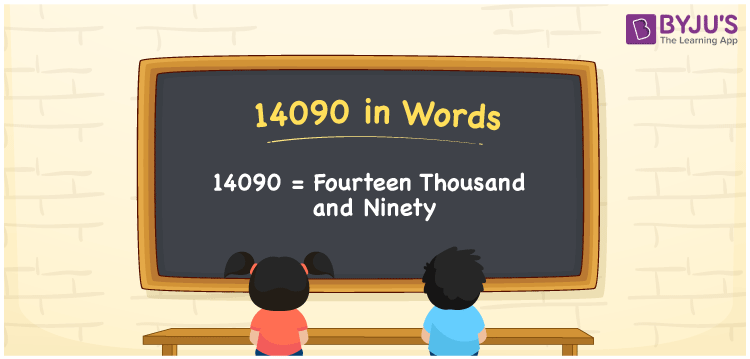# 14090 in Words

Fourteen Thousand and Ninety is the number name of 14090. Learning the number name is essential for everyone, as it helps to count the objects or to express the value of something. To write the number 14090 in words, the place value system is employed. Here is a detailed explanation of writing the number 14090 in words.

 14090 in Words: Fourteen Thousand and Ninety. Fourteen Thousand and Ninety in Numerical Form: 14090.

## 14090 in English Words## How to Write 14090 in Words?

The below table represents the place values of the 5-digit number 14090:

 Ten-thousands Thousands Hundreds Tens Ones 1 4 0 9 0

The expanded form of 14090 is as follows:

= 1 × Ten thousand + 4 × Thousand + 0 × Hundred + 9 × Ten + 0 × One

= 1 × 10000 + 4 × 1000 + 0 × 100 + 9 × 10 + 0 × 1

= 10000 + 4000 + 90

= 14090

= fourteen thousand and ninety

Hence, 14090 in words is fourteen thousand and ninety.

14090 in words – Fourteen thousand and ninety

Is 14090 an odd number? – No

Is 14090 an even number? – Yes

Is 14090 a perfect square number? – No

Is 14090 a perfect cube number? – No

Is 14090 a prime number? – No

Is 14090 a composite number? – Yes

## Frequently Asked Questions on 14090 in Words

Q1

### How to spell 14090 in English words?

14090 in words is fourteen thousand and ninety.

Q2

### Simplify 14000 + 90, and express it in words.

Simplifying 14000 + 90, we get 14090. Hence, 14090 in words is fourteen thousand and ninety.

Q3

### Is 14090 a prime number?

No, 14090 is not a prime number.